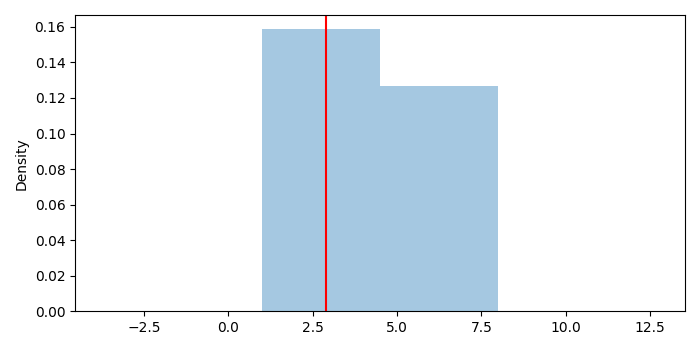# How to add vertical lines to a distribution plot (sns.distplot) in Matplotlib?

To add vertical lines to a distribution plot, we can take the following steps−

• Create a list of numbers.
• Create an axis using sns.displot().
• Get x and y data of the axis ax.
• Plot a vertical line on the plot.
• Remove the line at the 0th index.
• To display the figure, use show() method.

## Example

import seaborn as sns, numpy as np
import matplotlib.pyplot as plt
plt.rcParams["figure.figsize"] = [7.00, 3.50]
plt.rcParams["figure.autolayout"] = True
x = [5, 6, 7, 2, 3, 4, 1, 8, 2]
ax = sns.distplot(x, kde=True)
x = ax.lines.get_xdata()
y = ax.lines.get_ydata()
plt.axvline(x[np.argmax(y)], color='red')
ax.lines.remove()
plt.show()

## Output